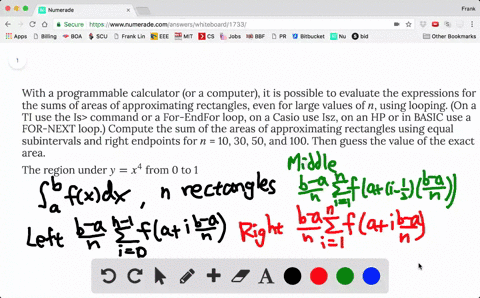### With a programmable calculator (or a computer), i…

01:44
FL
Problem 8

Evaluate the upper and lower sums for $f(x) = 1 + x^2$, $-1 \le x \le 1$, with $n$ = 3 and 4. Illustrate with diagrams like Figure 14.

$\begin{array}{ll}{n=3 :} & {\text { lower } \approx 2.148, \quad \text { upper } \approx 3.407} \\ {n=4 :} & {\text { lower }=2.25, \quad \text { upper }=3.25}\end{array}$

## Discussion

You must be signed in to discuss.

## Video Transcript

evil upper someone or some. So we first right arch and each interval and the fine the largest and smallest value at at AA for F they on the interval. So here are our use. And it was forcing example So our intervals from native one to one. So if we split into fine tomorrow, this should be minus one over two zero one over two. So what this Offer Someone lower, son. So upper sum for each interval, we want to pick the largest one. So s O on this interval. No, this f off negative ones. The largest one bills X Square has a small splatter here and largest larger value is away from zero. So on this interval for FC was one process where we have to pick half off. Negative one on this. Integral of all, For some reason, F s, um, is decreasing. So the upper one or is the largest value for F happened at def end point. This one efforts are increasing. So the largest one the point where F s largest value on this interval is f off one over two. The point on this interval for efforts the largest values there for one, it was again F is increasing for lower Some again, we have to pick the smallest f off F value on each interval. So here should be negative one over to because ofthis decreasing so the so the smallest. Why haven't right in point summer And this one should be f zero. This one should be half off zero and this one f it's increasing. So the small is the point. Wherever smallest values happens at left and point and each off each interval has based one over two. So the opera some would just be some off All these things you deprive by the base of the rock Yongle one over two and the lower some again will be some of these things community private, one over two, which is the pace of rectangle. And you can't do the similar things for sorcery